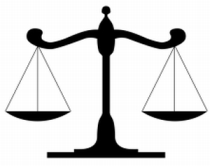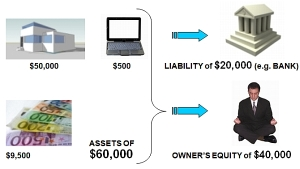# The Basic Accounting Equationor Formula

Previous lesson: Define Accounting
Next lesson: What Are Assets?

Check out the quiz in the Test Yourself! section further below when you're done with this lesson. And right at the bottom of the page, more questions on the topic submitted by fellow students.

Have you heard of the basic accounting equation or accounting formula?

Do you know what it is?

It's the fundamental equation that underpins all of accounting.

In other words, it's really important.

Why is it so important?

Because all accounting entries - all of them - are derived from it.

Let me say that again: All accounting entries. All of them.

So, if you really understand this equation, the rest of accounting becomes that much easier.

If you don't understand it, you'll probably find the subject very hard.

No pressure, right?

Okay, so pay attention...

The whole of accounting is based on one single equation:

﻿ASSETS = LIABILITIES + OWNERS EQUITY﻿The word equation comes from the word equal.

For any equation, one side always equals another.

Also, equations can actually be made out of anything.

For example:

• 1 Orange = \$0,50
• House = Walls + Doors + Windows + Roof
• 1 week = 7 days

So what does the basic accounting equation or basic accounting formula mean?

Well, in order to answer that question we need to look at what each of the terms in the equation mean.

## AssetsAssets are basically possessions of the business. They are things that add value to the business and will bring it benefits in some form.

For example, furniture, machinery, vehicles, computers, stationery or cash.

## LiabilitiesLiabilities are basically debts.

The amount of liabilities represents the value of the business assets that are owed to others. It is the value of the assets that people outside the business can lay claim to.

## Owners Equity (or Equity)Owners equity, or simply, equity, is the value of the business assets that the owner can lay claim to.

It is the value of the assets that the owner really owns.

## What the Basic Accounting Equation MeansIn a nutshell, the accounting equation above shows us:

• How much of the assets are owed to others (liabilities), and
• How much are owned by the owner (equity).

It's as simple as that.

## Test Yourself!

Before you start, I would recommend to time yourself to make sure that you not only get the questions right but are completing them at the right speed.

Difficulty Rating:
Beginner

Quiz length:
3 questions

Time limit:
5 minutes

Important: The solution sheet on the following page only shows the solutions and not whether you got each of the questions right or wrong. So before you start, get yourself a piece of paper and a pen to write down your answers. Once you're done with the quiz and writing down your answers, click the Check Your Answers button at the bottom and you'll be taken to our page of solutions.

Good luck!

## Basic Accounting Equation Mini Quiz:

Please note that all fields followed by an asterisk must be filled in.
 a) About half of accounting entries are based on the accounting equation. b) Only a few accounting entries are based on the accounting equation. c) Only accounting entries relating to assets are based on the accounting equation. d) All accounting entries are based on the accounting equation. e) None of the above.
 a) Liabilities. b) Owner's Equity. c) Possessions. d) Debts. e) None of the above.
 a) A form of owner's equity. b) Debts. c) Assets. d) Insurance. e) None of the above.

Please enter the word that you see below.

Does this equation and its meaning still seem a bit tricky right now? If so, don't worry, it will become easier as you continue along.

Read through the following lessons, where I will go into more details about each of the elements of the basic accounting equation, including examples for each one:

Once you are done with these lessons be sure to check out the final lesson on the accounting equation and financial position, which will give you more info and certainty about this key concept.

Did you know?

Not only does the accounting equation underpin all accounting entries, but it also forms the exact structure of one of accounting's most important reports - the balance sheet.

To see this report showing the accounting equation, check out the lesson on the balance sheet.

Stay up to date with ABfS!

Previous lesson: Define Accounting
Next lesson: What Are Assets?

### Questions Relating to This Lesson

Click below to see questions and exercises on this same topic from other visitors to this page... (if there is no published solution to the question/exercise, then try and solve it yourself)

Basic Accounting Equation Question
Before you begin: For purposes of exams and testing it's important to make sure you not only answer the questions correctly but are completing them …